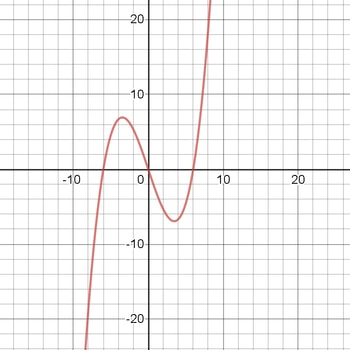# For the given expression y', find y'' and sketch the general shape of the graph of y = f(x). y' =...

## Question:

For the given expression {eq}y' {/eq}, find {eq}y'' {/eq} and sketch the general shape of the graph of {eq}y = f(x) {/eq}.

{eq}\displaystyle y' = \frac{x^2}{4} - 3 {/eq}

## Graphs:

In such questions finding y is easy but while sketching the graph of the function, we get a constant of integration, so we can only draw a general shape of the graph as mentioned in the question.

## Answer and Explanation:

We have :

{eq}\displaystyle y' = \frac{x^2}{4} - 3 {/eq}

For calculating y we have to take derivative of y' :

{eq}\displaystyle y'' = \frac{d}{dx}(y')= \frac{d}{dx}(\frac{x^2}{4} - 3) \\\displaystyle \boxed{y'' = \frac{x}{2}} \\ \\ {/eq}

Now for the function f(x) we have to integrate y' :

{eq}\displaystyle f(x) = \int y' dx \\\displaystyle f(x) = \int (\frac{x^2}{4} - 3) dx \\\displaystyle f(x) = \frac{x^3}{12} - 3x + c {/eq}

Where c is constant of integration and we can ignore this in drawing the general shape of f(x).

Below is the sketched graph of {eq}\displaystyle f(x) = \frac{x^3}{12} - 3x {/eq}#### Learn more about this topic:Graphs: Types, Examples & Functions

from High School Algebra II: Help and Review

Chapter 16 / Lesson 11
355K